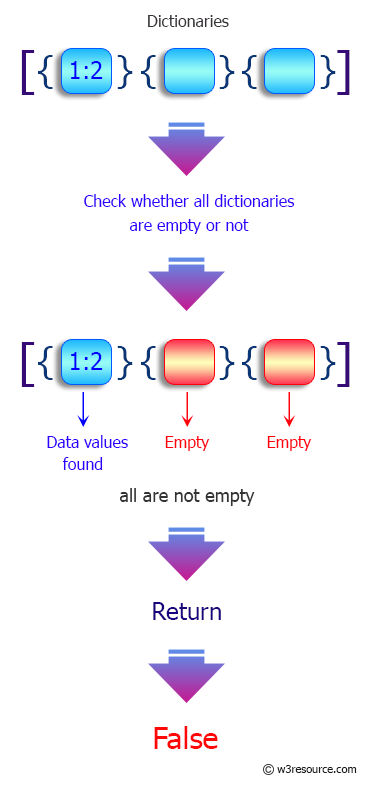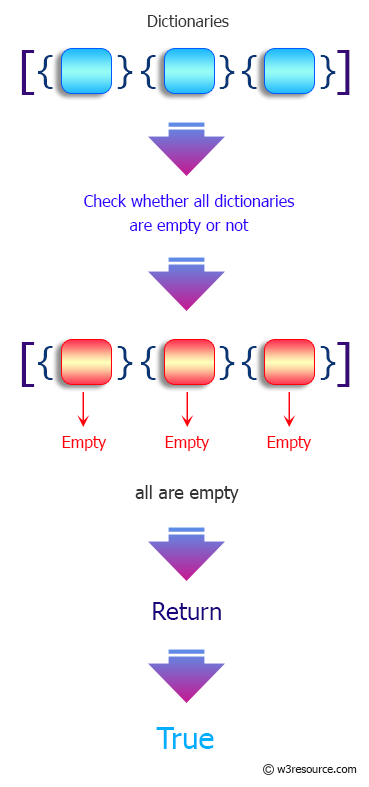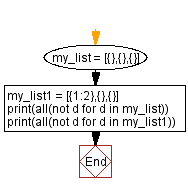﻿ Python: Check whether all dictionaries in a list are empty or not - w3resource

# Python: Check whether all dictionaries in a list are empty or not

## Python List: Exercise - 71 with Solution

Write a Python program to check whether all dictionaries in a list are empty or not.

Sample Solution:

Python Code:

``````my_list = [{},{},{}]
my_list1 = [{1:2},{},{}]
print(all(not d for d in my_list))
print(all(not d for d in my_list1))
```
```

Sample Output:

```True
False
```

Pictorial Presentation:Flowchart:## Visualize Python code execution:

The following tool visualize what the computer is doing step-by-step as it executes the said program:

Python Code Editor:

Have another way to solve this solution? Contribute your code (and comments) through Disqus.

What is the difficulty level of this exercise?

Test your Programming skills with w3resource's quiz.

﻿

## Python: Tips of the Day

Creates a list of elements, grouped based on the position in the original lists:

Example:

```def tips_zip(*args, fill_value=None):
max_length = max([len(lst) for lst in args])
result = []
for i in range(max_length):
result.append([
args[k][i] if i < len(args[k]) else fillvalue for k in range(len(args))
])
return result
print(tips_zip(['a', 'b'], [1, 2], [True, False]))
print(tips_zip(['a'], [1, 2], [True, False]))
print(tips_zip(['a'], [1, 2], [True, False], fill_value = '1'))
```

Output:

```[['a', 1, True], ['b', 2, False]]
[['a', 1, True], [None, 2, False]]
[['a', 1, True], ['1', 2, False]]
```